## 1. Introduction

In the context of a neural network, a neuron is the most fundamental unit of processing. It’s also called a perceptron. A neural network is based on the way a human brain works. So, we can say that it simulates the way the biological neurons signal to one another.

In this tutorial, we’ll study the neuron from both the biological context and the artificial neural network context.

## 2. Neural Network Terminology

Typically, from the biological perspective, we find neurons as part of the central nervous system and the human brain.

Apart from the living world, in the realm of Computer Science’s Artificial Neural Networks, a neuron is a collection of a set of inputs, a set of weights, and an activation function. It translates these inputs into a single output. Another layer of neurons picks this output as its input and this goes on and on. In essence, we can say that each neuron is a mathematical function that closely simulates the functioning of a biological neuron.

The following figure shows a typical neural network: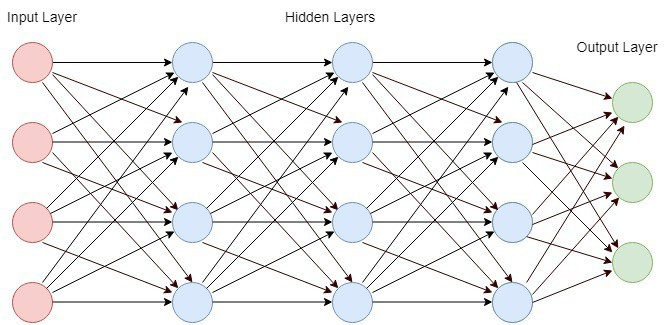## 3. Understanding Neurons

With this background, we now move on to study both the biological neuron and artificial neuron in greater depth.

### 3.1. Biological Neuron

We can define neurons as the information carriers that use electrical impulses and chemical signals to transmit information. The neurons transmit the information in the following two areas:

1. different parts of the brain
2. the brain and the nervous system

Thus, whatever we think, feel, and later do is all due to the working of the neurons.

The following figure shows a typical biological neuron: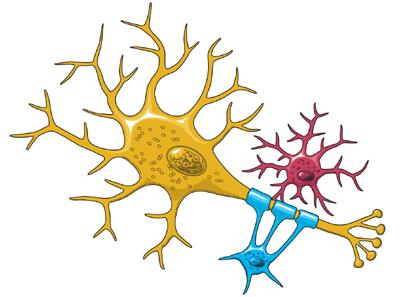Let’s briefly review the architecture of a neuron. A neuron has the following three basic parts:

1. Cell body
2. Cell extension Axon
3. Cell extension Dendrite

The following figure shows the architecture of a biological neuron: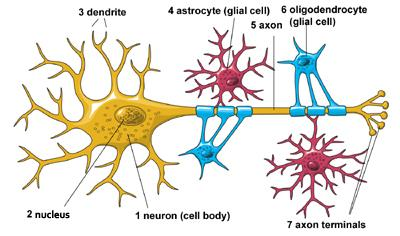The nucleus in the cell body controls the cell’s functioning. The axon extension (having a long tail) transmits messages from the cell. Dendrites extension (like a tree branch) receive messages for the cell.

So, in a nutshell, we can summarize that the biological neurons communicate with each other by sending chemicals, called neurotransmitters, across a tiny space, called a synapse, between the axons and dendrites of adjacent neurons.

### 3.2. Neuron in an Artificial Neural Network

After going through the biological neuron, let’s move to the artificial neuron.

An artificial neuron or neural node is a mathematical model. In most cases, it computes the weighted average of its input and then applies a bias to it. Post that, it passes this resultant term through an activation function. This activation function is a nonlinear function such as the sigmoid function that accepts a linear input and gives a nonlinear output.

The following figure shows a typical artificial neuron: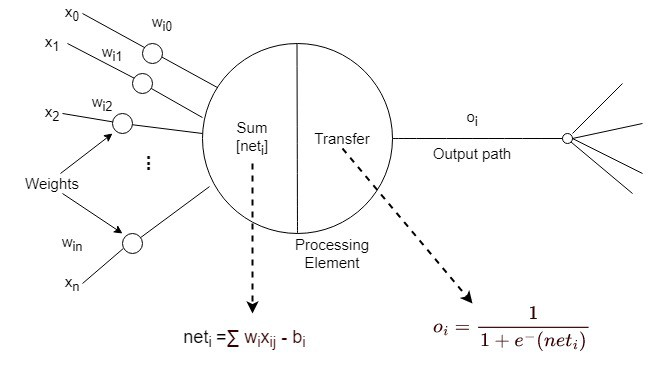## 4. Neurons and Neural Networks

A typical neural network consists of layers of neurons called neural nodes. These layers are of the following three types:

1. input layer (single)
2. hidden layer (one or more than one)
3. output layer (single)

Each neural node is connected to another and is characterized by its weight and a threshold. It gets an input on which it does some transformation and post that, it sends an output. If the output of any individual node is above the specified threshold value, that node gets activated. Then, it sends data to the next layer of the network. Otherwise, it remains dormant and thus doesn’t transmit any data to the next layer of the network.

The following figure marks all three types of layers: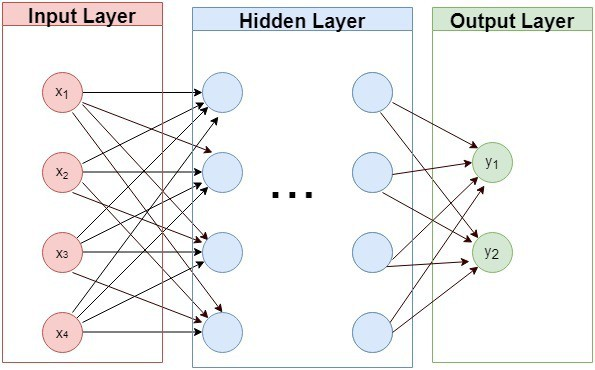Now, let’s look at each layer type of the neural network.

### 4.1. Input Layer

This is the first layer in a typical neural network. Input layer neurons receive the input information, process it through a mathematical function (activation function), and transmit output to the next layer’s neurons based on comparison with a preset threshold value. We usually have only one input layer in the network.

We pre-process text, image, audio, video, and other types of data to derive their numeric representation. Later, we pass this number representation as information to each input layer neuron. Each neuron then applies a predefined nonlinear mathematical function to calculate the output.

As a final step, we scale the output by preset weights linked to edges between the outgoing layer’s neurons and the incoming layer’s respective neurons.

### 4.2. Hidden Layer

Then comes the hidden layer. There can be one or more hidden layers in a neural network. Neurons in a hidden layer receive their inputs either from the neurons of the input layer or from the neurons of the previously hidden layer. Each neuron then passes the input to another nonlinear activation function and post that, it sends the output to the next layer neurons.

Here also, we multiply the data by edge weights as it is transmitted to the next layer.

### 4.3. Output Layer

At last, comes the output layer. We have only one output layer in the network that marks the logical ending of the neural network.

Similar to previously described layers, neurons in the output layer receive inputs from previous layers. Then, they process them through new activation functions and output the expected results. Depending on the type of Artificial Neural Network, we can either use this output as a final result or feed it as an input to the same neural network (loopback) or another neural network (cascade).

Now, let’s talk about the output layer’s result. We can have the final result as a simple binary classification denoting one of the two classes or we can have multi-class classifications. We can also use the final result as a predicted value.

Further, depending on the type of Artificial Neural Network, the final output could be used as a final result, or as an output to a new loop over the same or another neural net.

## 5. Types of Neural Networks

Here, we’ll study different types of neural networks based on the direction of information flow.

### 5.1. Feedforward Neural Network

Let’s first discuss the feedforward neural network.

Here, we find that the signal or the information travels in one way only. In a feedforward neural network, information flow starts from the input layer to the hidden layer(s) and finally to the output layer. We find no feedback or loops in it.

In other words, we can say that the output of a layer saydoes not affectin any way in this type of network. But, the output ofwill affect the output of the layers ahead of it. Feedforward neural networks are simple and straight networks. They have a one-to-one mapping between inputs with outputs.

The following figure shows a typical feedforward neural network: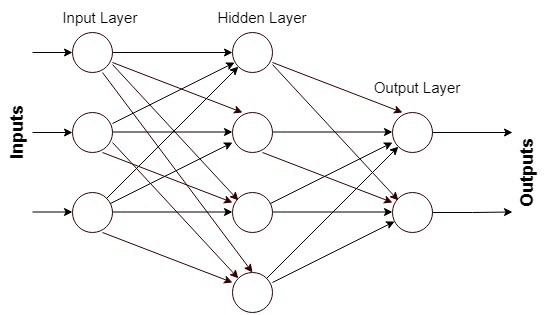We mostly use them in pattern generation, pattern recognition, and classification.

### 5.2. Feedback Neural Network

After going through the feedforward neural network, let’s move to the feedback neural network.

In this type of network, the signal or the information flows in both directions, i.e., forward and backward. This makes them more powerful and more complex than the feed-forward neural networks.

Feedback neural networks are dynamic because the network state keeps on changing until it reaches an equilibrium point. They remain at the equilibrium point till the input remains the same. Once the input changes, this process goes on until they find a new equilibrium.

We also call these networks interactive or recurrent networks due to their dynamic architecture. Moreover, we find feedback loops in these networks.

The following figure shows a typical feed backward neural network: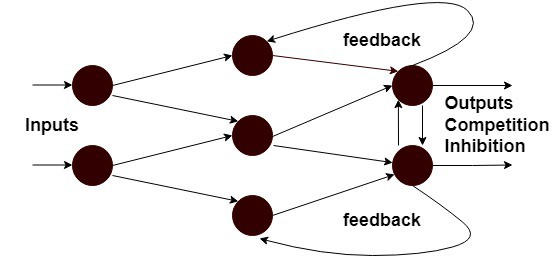We mostly use them in speech recognition, text prediction, and image recognition.

## 6. Benefits of Neural Networks

In this section, let’s enumerate the benefits of neural networks.

Neural networks possess the unique ability to derive quantifiable meaning from complicated or imprecise data. We can employ a well-structured neural network to extract patterns and detect otherwise too complex trends for us to discover and understand using other computer techniques.

Once we design a network for a specific problem and train it with a well-curated dataset, then we can analyze complex information using it. And this aids in decision-making at the highest level by providing projections for all plausible situations.

The single most significant benefit for neural networks is that they have adaptive learning capacity. We can understand this as the ability to learn how to do tasks based on the data given for training and then constantly improving its performance as it gets more and more data.

## 7. Conclusion

In this article, we studied the structure and functioning of artificial neurons. Further, we learned the architecture of a neural network, its layers, and its types and discussed their several important benefits.

We can conclude that just like the biological neuron, an artificial neuron is the battery of the underlying neural network, enabling it to function to perfection.